##### GRE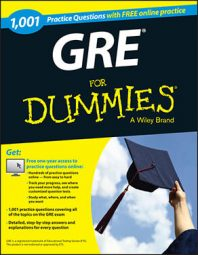The algebra problems on the GRE Math test may include linear equations, coordinate geometry, and quadratic equations. If you're fresh out of math class, you'll probably be fine going through these quickly.

If it's been a few years since you've done any algebra, you may want to work the questions carefully. Students who take the GRE tend to make the following mistakes:

• Mistakes in simple math, such as not placing the decimal point correctly

• Mistakes in working the problem, such as multiplying exponents when they should be adding them

• Not knowing how to work a certain math problem, such as setting up an equation

Here are some practice questions for you to try.

## Practice questions

1. Using the following figure,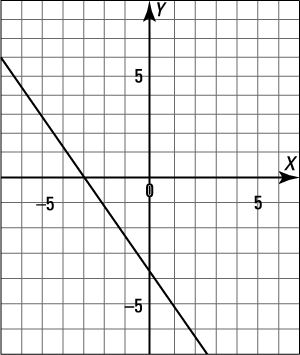find the equation of the line in this diagram.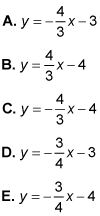2. The nth term of a sequence is given by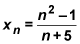Find the term of the sequence that is equal to 4.

A. The third term

B. The fifth term

C. The seventh term

D. The eighth term

E. The tenth term

1. C.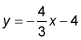First, find the slope: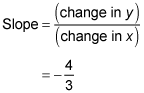Next, find the y-intercept, y-intercept = –4, for the line y = mx + b.

The equation for this line isThe correct answer is Choice (C).

2. C. The seventh term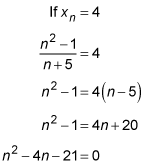This quadratic equation can be factored into (n – 7)(n + 3) = 0, which has solutions of n = 7 or n = –3.

Because n can't be negative, n = 7. The correct answer is therefore Choice (C).